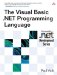# Exception Handling

 <  Day Day Up  >

Errors that occur while a program is running can be handled through the use of exceptions . An exception is an object derived from the class System.Exception that represents information about an exceptional situation (usually an error) that has occurred in a program. When an exceptional situation occurs, the program can throw an exception using the Throw statement. The Error statement can also be used to throw an exception based on an exception number instead of the exception type. The following code throws the same kind of exception (an invalid index was specified) using the two different statements.

` If x < 0 Then   Throw New IndexOutOfRangeException() End If If y < 0 Then   Error 9 End If `

Thrown exceptions can be caught by code that is designed to handle the error. Exceptions can be handled in one of two ways: unstructured exception handling or structured exception handling.

#### Structured Exception Handling

Structured exception handling allows exceptions to be handled for only a specific block of code. A Try statement handles any exceptions that occur when the statements contained within the Try statement are executed. A Try statement can specify Catch statements that can catch particular types of exceptions. A Catch statement can catch exceptions on the basis of either the type of the exception or a filter expression. In the following example, the function Divide handles a division-by-zero exception by printing an error message, while the Add function ignores exceptions based on the value of a parameter.

` Function Divide(ByVal x As Integer, ByVal y As Integer) As Integer   Try     Return x \ y   Catch e As DivideByZeroException     Console.WriteLine("Divide by zero.")   End Try End Function Function Add(ByVal x As Integer, ByVal y As Integer, _   ByVal IgnoreErrors As Boolean) As Integer   Try     Return x + y   Catch When IgnoreErrors     Console.WriteLine("Ignoring overflow.")   End Try End Function `

A Finally statement can be used to execute statements regardless of whether an exception was thrown inside a Try block or not. This ensures that the code is run even if an exception occurs.

` Sub DoWork(ByVal x As File)   Try     x.Open()     ...   Finally     ` Ensure file is closed even if an exception occurs     x.Close()   End Try End Sub `

#### Unstructured Exception Handling

Unstructured exception handling works by using On Error statements to establish the "current" exception handler. When an exception occurs, On Error GoTo can be used to direct execution to a label. On Error Resume Next can be used to direct execution to the next line after the one that caused the exception. In the following example, the Divide function handles a division-by-zero exception by printing an error message, while the Add function handles all exceptions by continuing to execute.

` Function Divide(ByVal x As Integer, ByVal y As Integer) As Integer   On Error GoTo HandleError   Return x \ y HandleError:   If Err.Number = 11 Then     Console.WriteLine("Divide by zero.")   End If End Function Function Add(ByVal x As Integer, ByVal y As Integer) As Integer   On Error Resume Next   Return x + y End Function `
 <  Day Day Up  >The Visual Basic .NET Programming Language
ISBN: 0321169514
EAN: 2147483647
Year: 2004
Pages: 173
Authors: Paul Vick

Similar book on Amazon